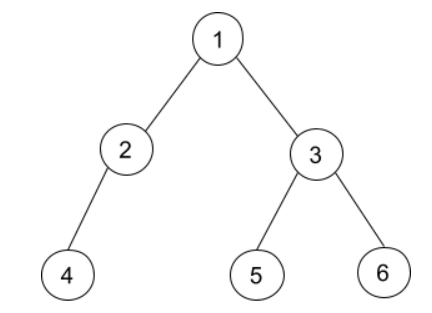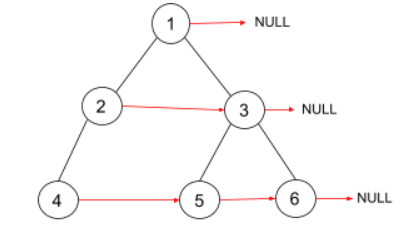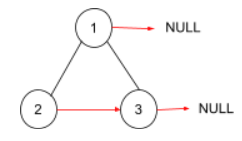Problem title
Difficulty
Avg time to solve

Kth factor of a number
Moderate
10 mins
Traffic Lights
Moderate
15 mins
Count Integers
Moderate
35 mins
Count Ways To Make Array With Product
Moderate
30 mins
Count Messages
Easy
15 mins
Restaurant Customers
Moderate
15 mins
Similar RGB Color
Easy
15 mins
Ninja And Movie Dilemma
Moderate
15 mins
Longest Subarray With No Repetitions
Moderate
25 mins
Ninja And The Nested Ranges
Moderate
25 mins1

# Populating Next Right Pointers in Each Node II

Difficulty: MEDIUM
Avg. time to solve
20 min
Success Rate
85%

Problem Statement

#### Note:

``````1. Node ‘U’ is said to be the next node of ‘V’ if and only if  ‘U’ is just next to ‘V’ in tree representation.
2. If there is no next right node, then the next pointer has to be ‘NULL’. Particularly root node and rightmost nodes have ‘next’ node equal to ‘NULL’.
3. Each node of the binary tree has three-pointers, ‘left’, ‘right’, and ‘next’. Here ‘left’ and ‘right’ are the children of a node, and ‘next’ is one extra pointer that we need to update.
``````
##### Input Format :
``````The first line contains an integer 'T' which denotes the number of test cases to be run.

The first line of each test case contains elements of the tree in the level order form. The line consists of values of nodes separated by a single space. In case a node is null, we take -1 in its place.

For example, the input for the tree is depicted in the below image.
````````````For example, the input for the tree depicted in the above image would be :

1
2 3
4 -1 5 6
-1 -1 -1 -1 -1 -1
``````

#### Explanation :

``````Level 1 :
The root node of the tree is 1

Level 2 :
Left child of 1 = 2
Right child of 1 = 3

Level 3 :
Left child of 2 = 4
Right child of 2 = null (-1)
Left child of 3 = 5
Right child of 3 =  6

Level 4 :
Left child of 4 = null (-1)
Right child of 4 = null (-1)
Left child of 5 = null (-1)
Right child of 5 = null (-1)
Left child of 6 = null (-1)
Right child of 6 = null (-1)
``````

#### Note :

``````The above format was just to provide clarity on how the input is formed for a given tree.
The sequence will be put together in a single line separated by a single space. Hence, for the above-depicted tree, the input will be given as:
1 2 3 4 -1 5 6 -1 -1 -1 -1 -1 -1
``````

#### Output Format :

``````For each test case, print the tree’s level order traversal after updating the ‘next’ for each node where  ‘-1’   denoting the null node.

The output of each test case will be printed in a separate line.
``````
##### Note :
``````You do not need to print anything. It has already been taken care of. Just implement the given function.
``````

#### Constraints :

``````1 <= T <= 5
0 <= N <= 3000
1 <= data <= 3000

Where ‘data’ is the value of the node of the binary tree.

Time Limit : 1 sec
``````
##### Sample Input 1 :
``````1
1 2 3 4 -1 5 6 -1 -1 -1 -1 -1 -1
``````
##### Sample Output 1 :
``````1 -1 2 3 -1 4 5 6 -1
``````
##### Explanation For Sample Input 1 :
``````The tree will be
``````##### Sample Input 2 :
``````1
1 2 3 -1 -1 -1 -1
``````
##### Sample Output 2 :
``````1 -1 2 3 -1
``````
##### Explanation For Sample Input 2 :
``````The tree will be:
``````Console